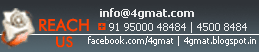Home GMAT Classes GMAT eBooks Math Books Practice Test Forums B Schools About Us
 GMAT Math eBooks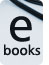Easy to download Math eBooks on select high scoring topics. Number Theory, Inequalities, Permutation, Probability, Geometry. \$6.99 eachGMAT Math Books & Workbooks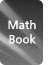Lesson Books for GMAT Math. Set of 5 books. Formulae, examples, exercises, shortcuts, tests. Rs.1650 within India. US \$89 outside India.GMAT Classes @ Chennai, Mumbai & Bangalore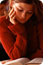Intensive classes for GMAT in Madras, Bombay, and Bangalore, India. 12 weekends, 72 hours. Classes on GMAT Math, Verbal and AWA. Includes study material and 10 CBTs.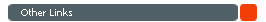Doubts, discussions in GMAT Math Share your GMAT Test experience 4GMAT's Achievers' List Testimonials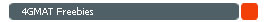Free GMAT Questions Answers Free GMAT Math Test (Quant Quiz) GMAT Blogs GMAT FAQs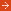Free Math Quiz - 4gmat.com4gmat.com presents a free math quiz and free sentence correction quiz that are updated periodically. The math quiz comprises interesting questions in Math that are representative of the questions that feature in the actual GMAT CAT test. Each quiz comprises 10 questions and are not timed. You can answer the questions at your pace and check your scores and evaluate your performance with the answer key provided. The questions are selected from a wide range of topics that feature in the GMAT Math section that include the following sections viz., Arithmetic, Algebra, Geometry. Topics covered include number theory, permutation and combination, probability and word problems. Ideally, you should be able to finish the test within 15 minutes. However, as it is only a practice exercise try and solve all the problems even if it takes you more than 15 minutes. The sentence correction quiz comprises 10 sentence correction questions. A GMAT test taker should be able to finish the test in about 12 to 15 minutes. Latest Free GMAT Math Quiz » Archive of Past Free GMAT Math Quiz.